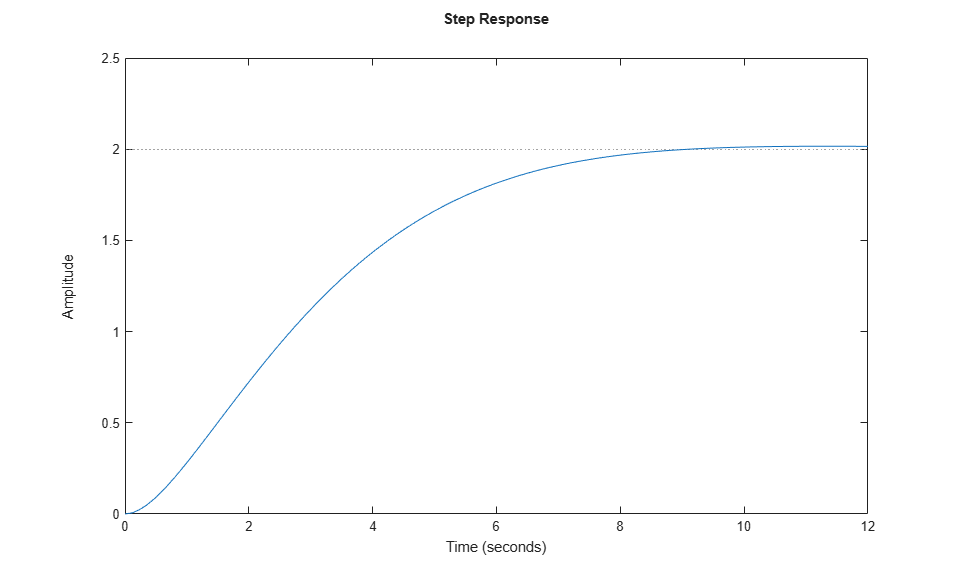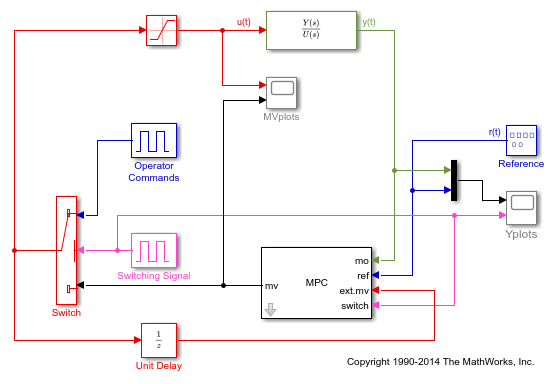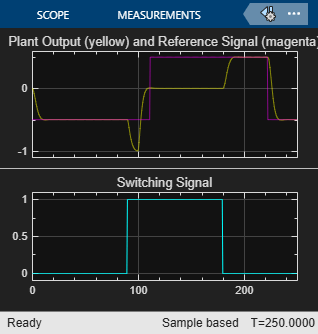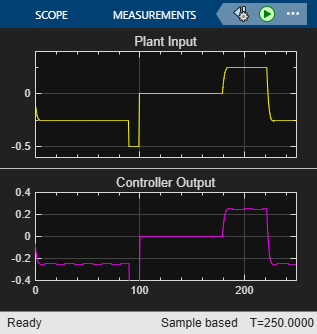Documentation

### This is machine translation

Mouseover text to see original. Click the button below to return to the English version of the page.

## Switching Controller Online and Offline with Bumpless Transfer

This example shows how to obtain bumpless transfer when switching model predictive controller from manual to automatic operation or vice versa.

In particular, it shows how the EXT.MV input signal to the MPC block can be used to keep the internal MPC state up to date when the operator or another controller is in control.

### Define Plant Model

The linear open-loop dynamic plant model is as follows:

```num = [1 1]; den = [1 3 2 0.5]; sys = tf(num,den); ```

### Design MPC Controller

Create an MPC controller with plant model, sample time and horizons.

```Ts = 0.5; % Sampling time p = 15; % Prediction horizon m = 2; % Control horizon mpcobj = mpc(sys,Ts,p,m); ```
```-->The "Weights.ManipulatedVariables" property of "mpc" object is empty. Assuming default 0.00000. -->The "Weights.ManipulatedVariablesRate" property of "mpc" object is empty. Assuming default 0.10000. -->The "Weights.OutputVariables" property of "mpc" object is empty. Assuming default 1.00000. ```

Define constraints on the manipulated variable.

```mpcobj.MV=struct('Min',-1,'Max',1); ```

Change the output weight.

```mpcobj.Weights.Output=0.01; ```

To achieve bumpless transfer, the initial states of your plant and controller must be the same, which is the case for the plant and controller in this example. However, if the initial conditions for your system do not match, you can set the initial states of the controller to the plant initial states. To do so, extract the `mpcstate` object from your controller and set the initial state of the plant.

```stateobj = mpcstate(MPC1); stateobj.Plant = x0;```

where `x0` is a vector of the initial plant states. Then, in the MPC Controller block dialog, set the Initial Controller State to `stateobj`.

To run this example, Simulink® is required.

```if ~mpcchecktoolboxinstalled('simulink') disp('Simulink(R) is required to run this example.') return end ```

Simulate closed-loop control of the linear plant model in Simulink. In this model, in the MPC Controller block dialog, the MPC Controller property is specified as `mpcobj`.

```mdl = 'mpc_bumpless'; open_system(mdl) sim(mdl) ```
```-->Converting the "Model.Plant" property of "mpc" object to state-space. -->Converting model to discrete time. -->Assuming output disturbance added to measured output channel #1 is integrated white noise. -->The "Model.Noise" property of the "mpc" object is empty. Assuming white noise on each measured output channel. ```### Simulate without Using External MV Signal

Without using the external MV signal, MPC controller is no longer able to provide bumpless transfer because the internal controller states are not estimated correctly.

```delete_line(mdl,'Switch/1','Unit Delay/1'); delete_line(mdl,'Unit Delay/1','MPC Controller/3'); delete_block([mdl '/Unit Delay']); delete_line(mdl,'Switching/1','MPC Controller/4'); set_param([mdl '/MPC Controller'],'mv_inport','off'); set_param([mdl '/MPC Controller'],'switch_inport','off'); set_param([mdl '/Yplots'],'Ymin','-1~-0.1') set_param([mdl '/Yplots'],'Ymax','3~1.1') set_param([mdl '/MVplots'],'Ymin','-1.1~-5') set_param([mdl '/MVplots'],'Ymax','1.1~10') sim(mdl) ```Now the transition from manual to automatic control is much less smooth. Note the large "bump" between time = 180 and 200.

```bdclose(mdl) ```